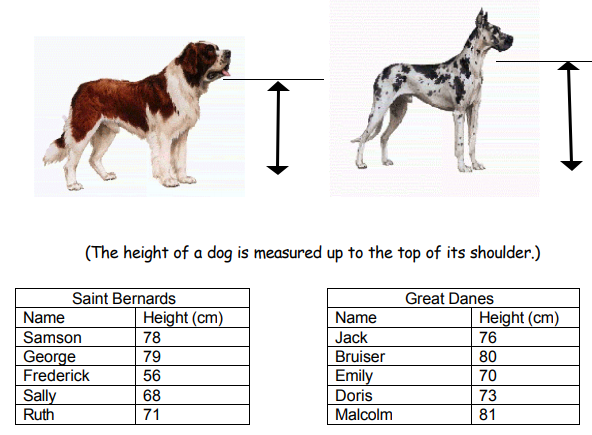Subject:
Mathematics

Topics: Statistics
Common Core State Standard: 6.SP.2, 6.SP.3, 6.SP.5c, 7.SP.1, S-ID.2,
Concepts:

· Average
· Range
· Mode

Knowledge and Skills:

· Knows the purpose of the subject of statistics
· Can find the mean, mode and range of a set of numbers

Lesson:

Procedure: Pose this question for open-ended class discussion: Ask students to state which type of dog they think is bigger, and (most importantly) to explain why they say so.

Help students understand that what makes it difficult to answer this question is that there are so many numbers to look at, and tell them that mathematicians have tried to solve this kind of problem by finding simple ways to talk about a whole group of numbers related to some particular situation, and this branch of mathematics is called “statistics”.

Review the definition of “statistics” and ensure that students grasp and can give examples of each part of it: collecting numerical information, organizing numerical information, summarizing numerical information, and explaining the meaning of numerical information.

With regards to the last point, you may want to discuss how people can have different explanations about the meaning of numerical information, depending on what they want you to believe.

Then, with simple examples, make sure that students understand “range,” average,” and “mode”. They should grasp that these are all different methods of summarizing numerical information.

If you wish, compute these measures for the dogs as a class. In this discussion, ask students to compare the measures (“How is average different from mode?,” “What does the range tell you that the average doesn’t tell you?,” etc.)

### The Tallest Dog

Which is bigger, a Saint Bernard or a Great Dane? You can figure out the answer to this question with the following information provided by veterinarian about some of her patients in each breed.How would you decide which type of dog is taller?

Words:

Statistics: Collecting, organizing, summarizing and explaining the meaning of numerical information.

Range: In a group of numbers, the difference between the lowest number and the highest number.

Average: The result of adding together a group of numbers and dividing by how many numbers there are.

Mode: The number that appears most often in a group of numbers.